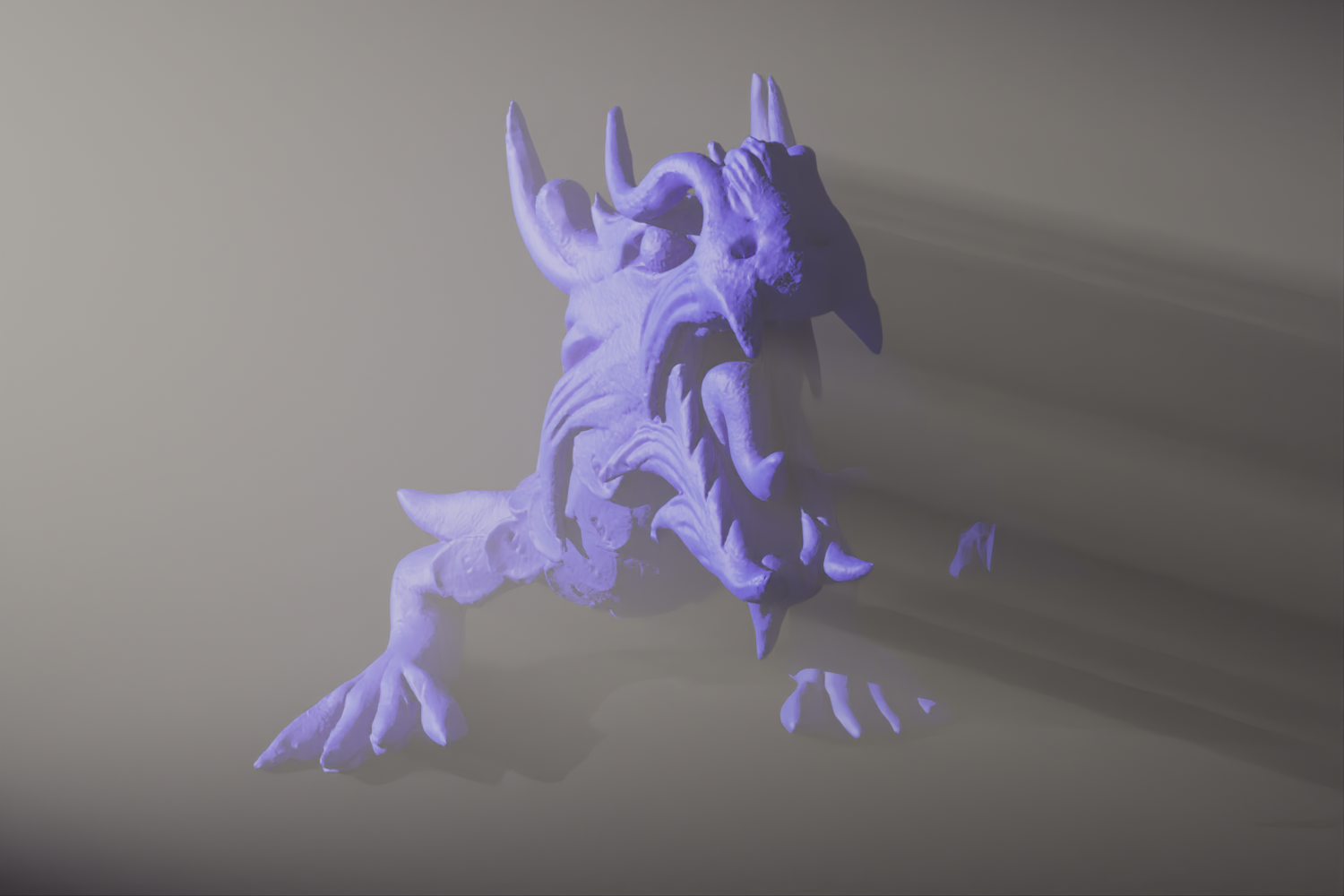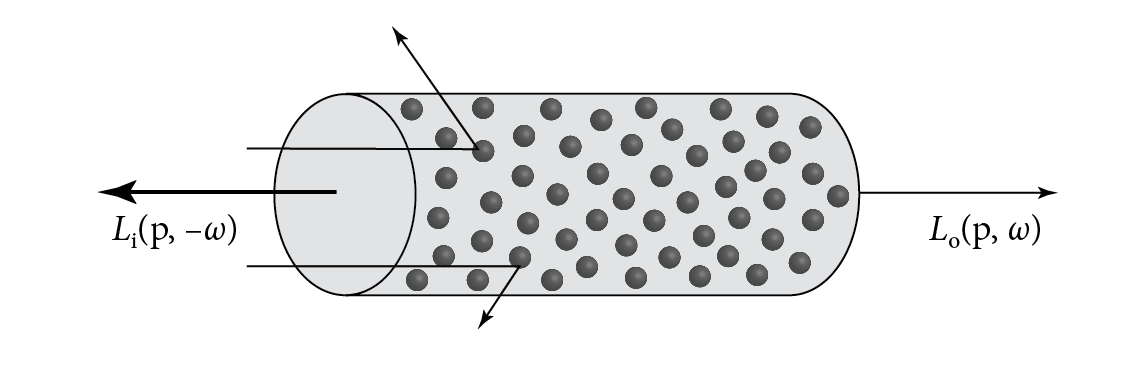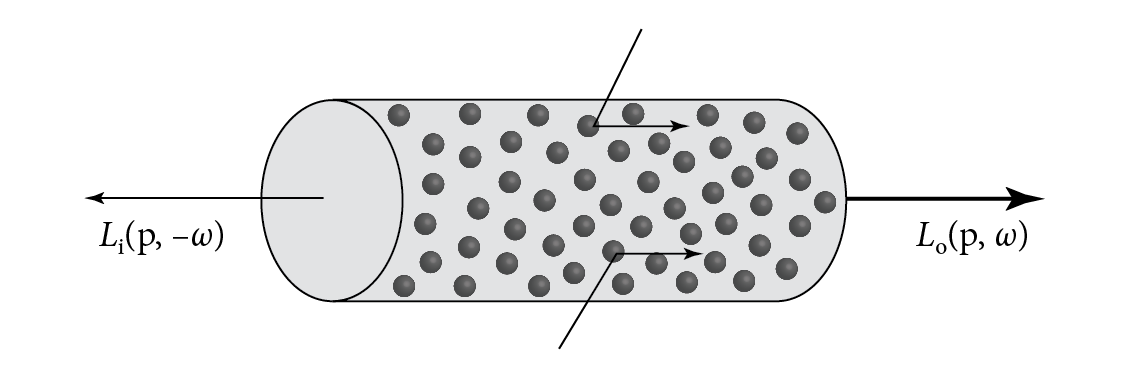## 11.1 Volume Scattering Processes

There are three main physical processes that affect the distribution of radiance in an environment with participating media:

• Absorption: the reduction in radiance due to the conversion of light to another form of energy, such as heat.
• Emission: radiance that is added to the environment from luminous particles.
• Scattering: radiance heading in one direction that is scattered to other directions due to collisions with particles.

The characteristics of all of these properties may be homogeneous or inhomogeneous. Homogeneous properties are constant throughout some region of space, while inhomogeneous properties vary throughout space. Figure 11.1 shows a simple example of volume scattering, where a spotlight shining through a homogeneous participating medium illuminates particles in the medium and casts a volumetric shadow.All of these processes may have different behavior at different wavelengths of light. While wavelength-dependent emission can be handled in the same way that it is from surface emitters, wavelength-dependent absorption and scattering require special handling in Monte Carlo estimators. We will gloss past those details in this chapter, deferring discussion of them until Section 14.2.2.

Physically, these processes all happen discretely: a photon is absorbed by some particle or it is not. We will nevertheless model all of these as continuous processes, following the same assumptions as underlie our use of radiometry to model light in pbrt (Section 4.1). However, as we apply Monte Carlo to solve the integrals that describe this process, we will end up considering the effect of these processes at particular points in the scene, which we will term scattering events. Note that “scattering events” is a slight misnomer, since absorption is a possibility as well as scattering.

All the models in this chapter are based on the assumption that the positions of the particles are uncorrelated—in other words, that although their density may vary spatially, their positions are otherwise independent. (In the context of the colors of noise introduced in Section 8.1.6, the assumption is a white noise distribution of their positions.) This assumption does not hold for many types of physical media; for example, it is not possible for two particles to both be in the same point in space and so a true white noise distribution is not possible. See the “Further Reading” section at the end of the chapter for pointers to recent work in relaxing this assumption.

### 11.1.1 Absorption

Consider thick black smoke from a fire: the smoke obscures the objects behind it because its particles absorb light traveling from the object to the viewer. The thicker the smoke, the more light is absorbed. Figure 11.2 shows this effect with a realistic cloud model.

Absorption is described by the medium’s absorption coefficient, , which is the probability density that light is absorbed per unit distance traveled in the medium. (Note that the medium absorption is distinct from the absorption coefficient used in specifying indices of refraction of conductors, as introduced in Section 9.3.6.) It is usually a spectrally varying quantity, though we will neglect the implications of that detail in this chapter and return to them in Section 14.2.2. Its units are reciprocal distance (), which means that can take on any nonnegative value; it is not required to be between 0 and 1, for instance. In general, the absorption coefficient may vary with both position and direction , although the volume scattering code in pbrt models it as purely a function of position. We will therefore sometimes simplify notation by not including in the use of and other related scattering properties, though it is easy enough to reintroduce when it is relevant.

Figure 11.3 shows the effect of absorption along a very short segment of a ray. Some amount of radiance is arriving at point , and we would like to find the exitant radiance after absorption in the differential volume. This change in radiance along the differential ray length is described by the differential equation

which says that the differential reduction in radiance along the beam is a linear function of its initial radiance. (This is another instance of the linearity assumption in radiometry: the fraction of light absorbed does not vary based on the ray’s radiance, but is always a fixed fraction.)This differential equation can be solved to give the integral equation describing the total fraction of light absorbed for a ray. If we assume that the ray travels a distance in direction through the medium starting at point , the surviving portion of the original radiance is given by

### 11.1.2 Emission

While absorption reduces the amount of radiance along a ray as it passes through a medium, emission increases it due to chemical, thermal, or nuclear processes that convert energy into visible light. Figure 11.4 shows emission in a differential volume, where we denote emitted radiance added to a ray per unit distance at a point in direction by . Figure 11.5 shows the effect of emission with a data set from a physical simulation of an explosion.The differential equation that gives the change in radiance due to emission is

The presence of on the right hand side stems from the connection between how efficiently an object absorbs light and how efficiently it emits it, as was introduced in Section 4.4.1. That factor also ensures that the corresponding term has units of radiance when the differential equation is converted to an integral equation.

Note that this equation incorporates the assumption that the emitted light is not dependent on the incoming light . This is always true under the linear optics assumptions that pbrt is based on.

### 11.1.3 Out Scattering and Attenuation

The third basic light interaction in participating media is scattering. As a ray passes through a medium, it may collide with particles and be scattered in different directions. This has two effects on the total radiance that the beam carries. It reduces the radiance exiting a differential region of the beam because some of it is deflected to different directions. This effect is called out scattering (Figure 11.6) and is the topic of this section. However, radiance from other rays may be scattered into the path of the current ray; this in-scattering process is the subject of the next section. We will sometimes say that these two forms of scattering are real scattering, to distinguish them from null scattering, which will be introduced in Section 11.2.1.The probability of an out-scattering event occurring per unit distance is given by the scattering coefficient, . Similar to absorption, the reduction in radiance along a differential length due to out scattering is given by

The total reduction in radiance due to absorption and out scattering is given by the sum . This combined effect of absorption and out scattering is called attenuation or extinction. The sum of these two coefficients is denoted by the attenuation coefficient :

Two values related to the attenuation coefficient will be useful in the following. The first is the single-scattering albedo, which is defined as

Under the assumptions of radiometry, the single-scattering albedo is always between 0 and 1. It describes the probability of scattering (versus absorption) at a scattering event. The second is the mean free path, , which gives the average distance that a ray travels in a medium with attenuation coefficient before interacting with a particle.

### 11.1.4 In Scattering

While out scattering reduces radiance along a ray due to scattering in different directions, in scattering accounts for increased radiance due to scattering from other directions (Figure 11.7). Figure 11.8 shows the effect of in scattering with the cloud model. There is no absorption there, corresponding to a single scattering albedo of 1. Light thus scatters many times inside the cloud, giving it a very different appearance.Assuming that the separation between particles is at least a few times the lengths of their radii, it is possible to ignore inter-particle interactions when describing scattering at a particular location. Under this assumption, the phase function describes the angular distribution of scattered radiation at a point; it is the volumetric analog to the BSDF. The BSDF analogy is not exact, however. For example, phase functions have a normalization constraint: for all , the condition

must hold. This constraint means that phase functions are probability distributions for scattering in a particular direction.

The total added radiance per unit distance due to in scattering is given by the source function :

It accounts for both volume emission and in scattering:

The in-scattering portion of the source function is the product of the albedo and the amount of added radiance at a point, which is given by the spherical integral of the product of incident radiance and the phase function. Note that the source function is very similar to the scattering equation, Equation (4.14); the main difference is that there is no cosine term since the phase function operates on radiance rather than differential irradiance.